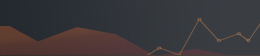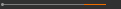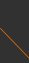Sponsored by[ Events ]

﻿
Activity Search
Sort out
 select all Seminars and Talks Conference and Workshop Lectures and Courses ElseField
 select all Number Theory and Representation Theory Algebraic Geometry Differential Geometry and Geometrical Analysis Differential Equations and Stochastic Analysis Scientific Computing Interdisciplinary Studies Laboratory of Data Science Harmonic Analysis OthersYear
 select 2015 2016 2017 2018 2019 2020 2021NCTS Seminar on Mathematical Biology10:00 - 12:00, April 10, 2020 (Friday)
Lecture Room B, 4th Floor, The 3rd General Building, NTHU
(清華大學綜合三館 4樓B演講室)
Cancelled--Introduction to Cauchy Problems with Non-dense Domain (II)
Feng-Bin Wang (Chang Gung University)

# This seminar is cancelled due to the severe situation caused by Chinese virus.

Abstract:

Mathematical models of population dynamics highly rely on the assumption of determinism. One of the assumptions is that the present state determines the future state, leading to ordinary or reaction-diffusion equations with initial conditions. Models for populations structured by age (age-structured population systems) are motivated by an alternative assumption that the past determines the future. The theory of linear semigroups of operators has played central roles in the study of age-structured population models. In such classical linear semigroup theory, the associated operator is assumed to be densely defined in the state space. Another powerful approach in investigating age-structured models is to rewrite it as an abstract Cauchy problem in which the associated operator may be nondensely defined and the non Hille-Yosida case. It is worth noting that functional differential equations, parabolic partial differential equations, and partial differential equations with delay, can be formulated as non-densely defined Cauchy problems. For the non-densely defined case, Pierre Magal and Shigui Ruan develop an extension of linear operator semigroup theory to the case that the semigroup has an integrated form, that is, integrated semigroups.
In a series of talks, I shall introduce the theory of integrated semigroups of operators and applications of non-densely defined Cauchy problems.

References:

W. Arendt, C. J. K. Batty, M. Hieber, and F. Neubrander, Vector-Valued
Laplace Transforms and Cauchy Problems (Second Edition), Birkhauser, Basel, 2010.
 P. Magal and S. Ruan, Theory and Applications of Abstract Semilinear
Cauchy Problems, Applied Mathematical Sciences, Springer, 2018.
 P. Magal, O. Seydi, F.-B. Wang, Monotone abstract non-densely defined Cauchy problems applied to age structured population dynamic models, J. Math. Anal. Appl. 479 (2019), 450-481.
A. Pazy, Semigroups of Linear Operator and Applications to Partial Differential Equations, Springer-Verlag, New York, 1983.
H. R. Thieme, "Integrated semigroups" and integrated solutions to abstract Cauchy problems, J. Math. Anal. Appl. 152 (1990), 416-447.(C) 2019 National Center for Theoretical Sciences